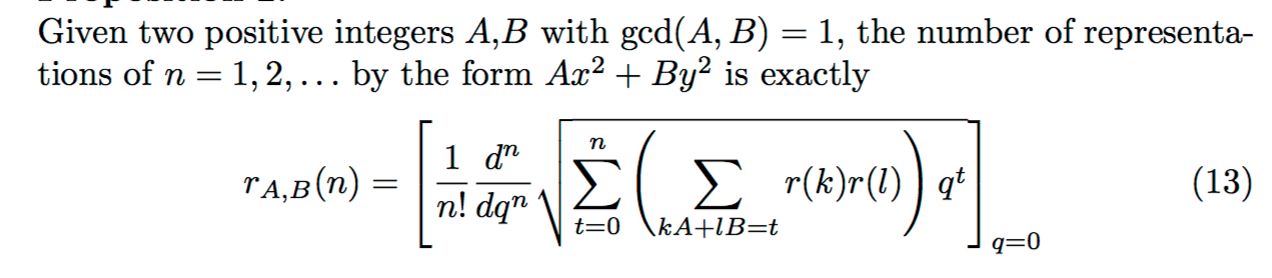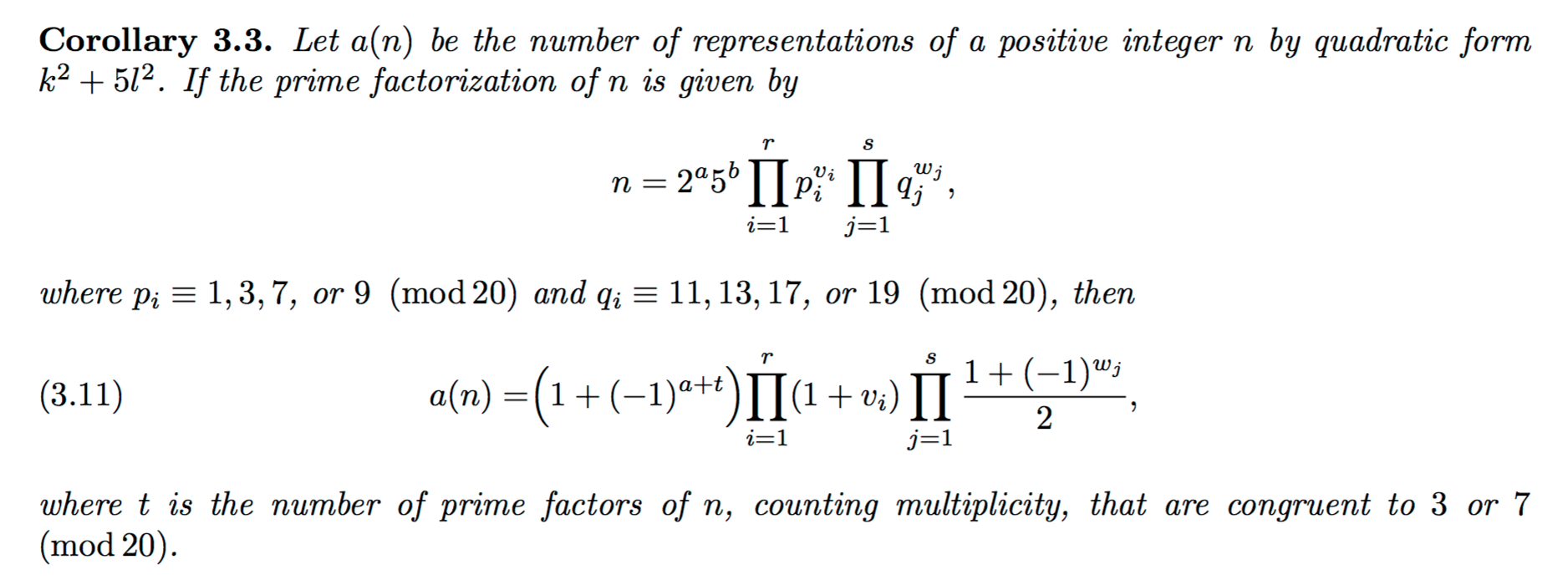# Jacobi's theorem on sums of two squares (reference request)

One of Jacobi's theorems states that the number of representations of a positive integer $n$ as a sum of two squares of integers equals $$4(d_1(n)-d_3(n)),$$ where the function $d_i$ counts the number of positive integer divisors congruent to $i \mod{4}$ of $n$.

My question is whether there are similar formulas for representations by the quadratic form $x^2+ay^2$, where $a$ is an integer other than $1$. If so, are there any references ?

Using class field theory one can prove for every integer $$n>0$$ there exists a monic polynomial $$f$$ of degree $$h(-4n)$$* such that for any odd integer $$m$$ coprime to $$n$$ we have the following equivalence:

$$\exists x,y\in\mathbb{Z}:(m=x^2+ny^2)\iff \exists x,y\in\mathbb{Z}:(f(x)\equiv 0\bmod m)\land (y^2\equiv-n\bmod m)$$

However depending on the polynomial $$f$$ this may or may not readily translate into a "nice" sum of Jacobi symbols, namely any expression counting solutions of this form is going to likewise need to count adjusted roots in $$f$$ modulo primes dividing $$m$$ which can then be lifted back to solutions with Henzels lemma and with the Chinese remainder theorem (even ignoring other problems). Though we can as I said find similar "nice" formulas for special cases e.g. the particular values of $$n$$ which force certain pdbqf's of discriminate $$D=-4n$$ to lie in the same genus, in particular when all the forms are in the same genus as the principal form i.e. they range over the same values in $$(\mathbb{Z}/D\mathbb{Z})^{\times}$$ we find that it is possible to fully characterize the primes $$p$$ with solutions $$x,y\in\mathbb{Z}$$ to $$p=x^2+ny^2$$ using only conditions on the residues of $$p$$ modulo fixed integers. For example we can show that:

$$\exists x,y\in\mathbb{Z}:p=x^2+6y^2\iff p\equiv 1, 7\bmod 24$$ $$\exists x,y\in\mathbb{Z}:p=x^2+21y^2\iff p\equiv 1,25,37\bmod 84$$ $$\exists x,y\in\mathbb{Z}:p=x^2+15y^2\iff p\equiv 1, 19, 31, 49\bmod 60$$

Though despite this the formula $$r_2(n)=4(d_1(n)-d_3(n))$$ can be generalized to counting proper representations of any integer $$n$$ by all the positive definite binary quadratic forms of an arbitrary fixed discriminant which can also be expressed as a similar divisor like sum involving Jacobi symbols using Dirichlet's Mass formula. While since the arbitrary representations of any $$n\in\mathbb{N}$$ by a quadratic form are proper representations of $$n/d$$ coordinate wise scaled by $$\sqrt{d}$$ for a square $$d\mid n$$. We can further prove that if $$r_2(n)=4(d_1(n)-d_3(n))$$ is the number of integer pairs $$(x,y)\in\mathbb{Z}^2$$ which satisfy $$x^2+y^2=n$$ whereas if $$s(n)=|\{d\mid n:\sqrt{d}\in\mathbb{N}\}|=\prod_{p\mid n}\left(1+\lfloor v_p(n)/2\rfloor\right)$$ is the number of perfect squares dividing $$n$$ and $$\omega(n)=\sum_{p\mid n}1$$ is the number of distinct primes dividing $$n$$ then if for every integer $$m$$ coprime to $$n$$ we define:

$$w(m)=\begin{cases}6&\text{ if }m=-3\\4&\text{ if }m=-4\\2&\text{ otherwise}\end{cases}$$

As well as the indicator function:

$$e_m(n)=\prod_{p\mid n}\frac{1}{2}\left(1+\left( \dfrac{m}{p}\right)\right)=\begin{cases}1&\text{ if }\exists x\in \mathbb{Z}:x^2\equiv m\bmod n\\0&\text{ if }\not\exists x\in \mathbb{Z}:x^2\equiv m\bmod n\end{cases}$$

We know if $$\mathscr{F}_D$$ is the set of reduced positive definite binary quadratic forms of discriminant $$D$$ so that by definition $$h(D)=|\mathscr{F}_D|$$ then for an arbitrary odd integer $$n\in\mathbb{N}$$ coprime to $$D$$ we must get that:

$$\small |\{(x,y,f)\in \mathbb{Z}^2\times\mathscr{F}_D:f(x,y)=n\land \gcd(x,y)=1\}|=w(D)\sum_{d\mid n}\left( \dfrac{D}{d}\right)=w(D)e_D(n)2^{\omega(n)}$$

$$\small\implies |\{(x,y,f)\in \mathbb{Z}^2\times\mathscr{F}_D:f(x,y)=n\}|=w(D)\sum_{d\mid n}\left( \dfrac{D}{d}\right)s(d)=w(D)e_D(n)(d_1(n)-d_3(n))$$

Thus in particular if $$D=-4$$ we get $$f(x,y)=x^2+y^2$$ is the only reduced form of discriminant $$D$$ so these formula simplify to your original identity for counting the number of representations of any integer as a sum of two squares. Whereas note if $$D=-28$$ then $$f(x,y)=x^2+7y^2$$ is the only reduced form of discriminant $$D$$ because $$h(-28)=1$$ therefore for every odd integer $$n>0$$ not divisible by $$7$$, when there exists integers $$a,b\in\mathbb{Z}$$ such that $$n=a^2+7b^2$$ then we must have by both of our previous formula that:

$$|\{(x,y)\in\mathbb{Z}^2:x^2+7y^2=n\land \gcd(x,y)=1\}|=2^{\omega(n)+1}$$ $$|\{(x,y)\in\mathbb{Z}^2:x^2+7y^2=n\}|=2(d_1(n)-d_3(n))$$

For details on reduction theory read Chapter 4 of this: http://www2.math.ou.edu/~kmartin/ntii/ntii.pdf Whereas for Dirichlet's formula read page 5 of this: http://www2.math.ou.edu/~kmartin/ntii/chap4.pdf For a more general approach that includes material discussed at the start, try this book by David Cox.

• The last equality is not correct. Do you mean to take the sum over squarefree $d|n$ ? Jun 23, 2016 at 16:07
• @CaptainDarling Sorry, I was counting the number of proper representations. I updated it. Jun 24, 2016 at 0:02

The requested generalization of Jacobi's two-square theorem is a remarkably recent result: N. Bagis and M.L Glasser, On the Number of Representations of Integers by various Quadratic and Higher Forms (2015):where $$r(n)$$ is Jacobi's formula for the number of representations of $$n=x^2+y^2$$.

I understand from the comments that this (general but complicated) formula is not what the OP was looking for. Berkovich and Yesilyurt in Ramanujan's Identities and Representation of Integers by Certain Binary and Quaternary Quadratic Forms (2006) give rather simple expressions for specific cases of $$n=x^2+ay^2$$. I reproduce below the result for $$a=5$$, and there are others (6,15,27,...). As explained in this 2012 MO posting, there is no simple Jacobi-type formula that will apply to any $$a$$.• I don't consider this formula to be "similar" to Jacobi's formula - it is way too unwieldy. In cases that the binary form $Q = x^2 + ay^2$ has class number $1$, there should be a formula equating the theta series of $Q$ with an Eisenstein series, and be very similar to Jacobi's formula. This would occur only for $a = 1, 2, 3$ and $7$, though. Jun 22, 2016 at 19:50
• the formula immediately generalizes to more than two squares, $n=\sum_{k=1}^N A_k x_k^2$, see equation 15 of the cited paper; I find it actually more memorable than unwieldy. Jun 22, 2016 at 20:37
• I agree with Jeremy Rouse. The quoted formula (13) expresses in analytic form the simple fact that $\sum_{m+n=t}r_{A,B}(m)r_{A,B}(n)=\sum_{kA+lB=t}r(k)r(l)$. Indeed, both sides count the number of quadruples $(u,v,x,y)\in\mathbb{Z}^4$ such that $Au^2+Bv^2+Ax^2+By^2=t$. In contrast, Jacobi's formula has to do with arithmetic, e.g. the fundamental theorem of arithmetic in $\mathbb{Z}[i]$. Jun 22, 2016 at 23:50

Several examples are in L. E. Dickson, Introduction to the Theory of Numbers. Jacobi's result is Theorem 65 on page 80, several forms of class number one are in the exercises pages 80-81. Several forms with one class per genus are exercises on pages 84-88, including a few odd discriminants.

Liouville published a series of papers concerning representations of a number by different quadratic forms. He gave many identities without proofs. Liouville's methods were reconstructed by Uspensky, see chapter "Liouville's methods" from the book Uspensky J. V., Heaslet M. A. Elementary number theory. McGraw-Hill New York, 1939.

One can also find another exposition of Liouville's methods in the survey Venkov B. A. Elementary number theory. Wolters-Noordhoff Publishing, Groningen, 1970.

• There's also Number Theory in the Spirit of Liouville, by Kenneth Williams, cambridge.org/core/books/… Jan 19, 2020 at 4:40